# 思考过程1. 初始化一个大小为（k+1）的数组C（所有元素初始值为0），遍历整个待排序数组A，将A中每个元素对应C中的元素大小+1。操作结果见下图：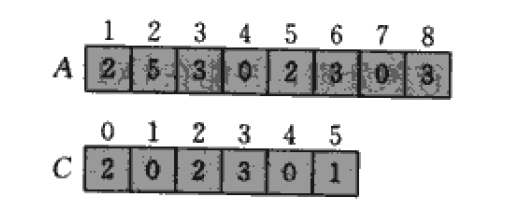2.我们将C中每个i位置的元素大小改成C数组前i项和（基于之前的算法思考，我们不难理解这么做的道理）：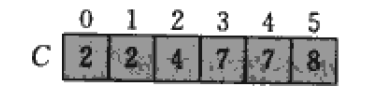3.OK，现在我们已经快看到成功的曙光了。现在要做的就是初始化一个和A同样大小的数组B用于存储排序后数组，然后倒序遍历A中元素（后面会提到为何要倒序遍历），通过查找C数组，将该元素放置到B中相应的位置，同时将C中对应的元素大小-1（表明已经放置了一个这样大小的元素，下次再放同样大小的元素，就要往前挤一个位置）。遍历完A数组后，就完成了所有的排序工作（只画出了前3步）：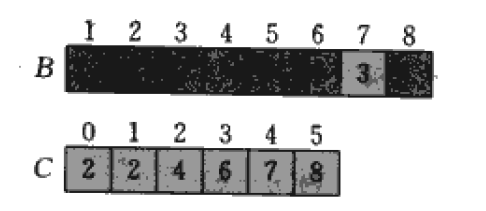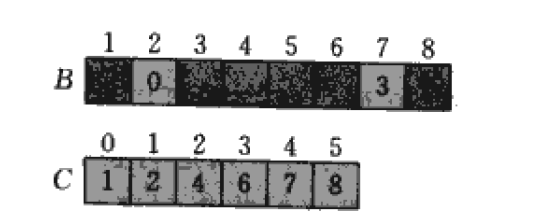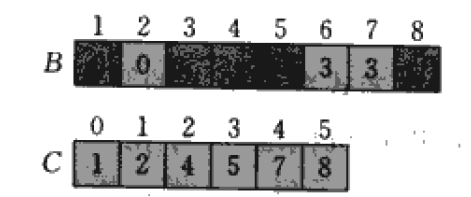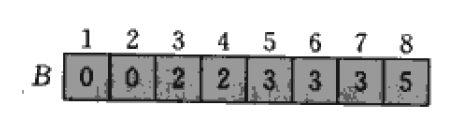# 最后附上计数排序的java代码：

package sort;
public class CountSort {

private static int[] countSort(int[] array,int k)
{
int[] C=new int[k+1];//构造C数组
int length=array.length,sum=0;//获取A数组大小用于构造B数组
int[] B=new int[length];//构造B数组
for(int i=0;i<length;i++)
{
C[array[i]]+=1;// 统计A中各元素个数，存入C数组
}
for(int i=0;i<k+1;i++)//修改C数组
{
sum+=C[i];
C[i]=sum;
}
for(int i=length-1;i>=0;i--)//遍历A数组，构造B数组
{

B[C[array[i]]-1]=array[i];//将A中该元素放到排序后数组B中指定的位置
C[array[i]]--;//将C中该元素-1，方便存放下一个同样大小的元素

}
return B;//将排序好的数组返回，完成排序

}
public static void main(String[] args)
{
int[] A=new int[]{2,5,3,0,2,3,0,3};
int[] B=countSort(A, 5);
for(int i=0;i<A.length;i++)
{
System.out.println((i+1)+"th:"+B[i]);
}
}
}

排序结果：posted on 2013-07-02 10:49  Leo-Yang  阅读(11240)  评论(6编辑  收藏  举报